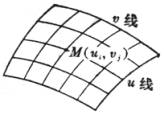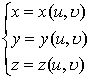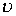§ 12      一般曲面图 7.23

F(x,y,z)=0

z=f(x,y)

参数式矢量式         r=r(u,)

r=x(u,)i+y(u,)j+z(u,)k